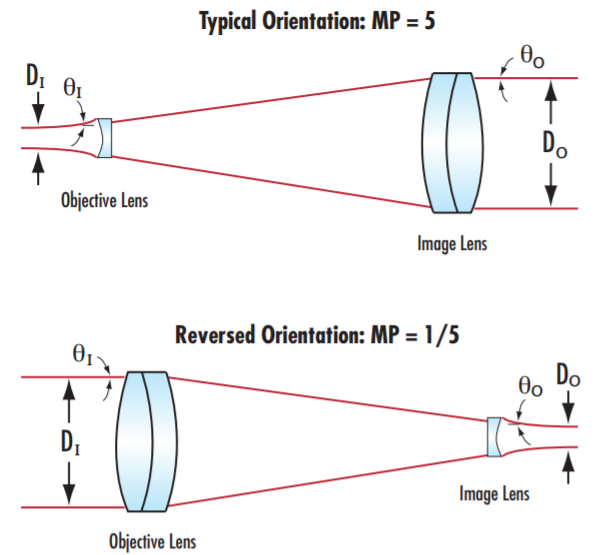# Can A Beam Expander be Used in Reverse?

Laser beam expanders can be used in reverse to reduce beam diameter rather than expanding it (Figure 1).##### Figure 1: Beam expanders typically use a small objective lens and a larger image lens to increase beam diameter, but they can be used in reverse with a large objective lens and a small image lens to decrease beam diameter.

Laser beam expanders are typically used to increase the diameter of a laser beam in order to decrease divergence, minimize focused spot size by expanding the beam before the focusing element, or prevent laser-induced damage inside a system by reducing power density. In a typical laser beam expander orientation, the beam will first encounter a small objective lens and then be collimated by a larger image lens (Figure 1). The relationships between beam diameter, divergence, and magnifying power (MP) are:

(1)$$\frac{\text{Input Beam Divergence} \left( \theta_I \right)}{\text{Output Beam Divergence} \left( \theta_O \right)} = \frac{\text{Output Beam Diameter} \left( D_O \right)}{\text{Input Beam Diameter} \left( D_I \right)}$$

The magnifying power (MP) can now be expressed in terms of the beam divergences or beam diameters.

(2)$$\text{MP} = \frac{\theta _I}{\theta _O}$$
(3)$$\text{MP} = \frac{D_O}{D_I}$$

Reversing the orientation of a laser beam expander will invert the magnifying power. For example, if a 5X laser beam expander is used in reverse, it will now have a new magnifying power of 1/5 instead of 5. The beam diameter is successfully reduced, but Equations 1 and 2 show that the divergence of the beam will increase. In the earlier example, a beam leaving the 5X laser beam expander will have an output beam divergence 1/5 of the input beam divergence. However, when used in reverse, the output beam divergence will be 5X that of the input beam divergence. This means that, in many cases, a laser beam expander used in reverse will reduce the beam diameter close to the laser beam expander, but divergence will cause the diameter to be larger at a distance. This may fail to achieve the user’s desired end result if their goal was to have a small beam at a distance.

The beam divergence of a laser can typically be found in a user manual or datasheet. It can also be approximated by measuring the beam diameter at a Distance 1 away from the laser, and again at Distance 2 away from the laser:

(4)$$\text{Laser Divergence } \left( \theta_L \right) = \theta_I = 2 \, \arctan{\left[ \frac{D_2 - D_1}{2 \left( \text{Distance between points 1 and 2} \right) } \right]}$$

There are other caveats to using a laser beam expander in reverse in addition to increased divergence because they are typically designed to magnify beams.

Ghost reflections - Typical laser beam expander designs take into account ghost reflections, or unwanted internal reflections off of lens elements, when they are being used to increase beam diameter. Unless the laser beam expander was specifically designed for a reverse orientation, it has likely not been verified for ghost reflections for reverse use. Ghost reflections can lead to internal focusing that can reduce beam quality and potentially damage internal elements. This is not particularly common because a laser beam expander with no ghost reflections used in the typical orientation will not have them in the reversed orientation. However, ghost reflections are still a possibility and so are worth verifying, especially when used with high power lasers.

Laser-induced damage threshold (LIDT) - Damage thresholds are typically specified as the maximum incident power or energy at the input aperture. When using the laser beam expander in reverse, what was the exit aperture is now the “new” input aperture. This surface now is exposed to higher energy than it typically would be, and this needs to be taken into consideration when verifying the power or energy handling capabilities of the laser beam expander.